## Example Questions

← Previous 1 3 4

### Example Question #1 : Quadratic Equation

Solve for x: x2 + 4x = 5

-5

-1

-5 or 1

-1 or 5

-5 or 1

Explanation:

Solve by factoring.  First get everything into the form Ax2 + Bx + C = 0:

x2 + 4x - 5 = 0

Then factor: (x + 5) (x - 1) = 0

Solve each multiple separately for 0:

X + 5 = 0; x = -5

x - 1 = 0; x = 1

Therefore, x is either -5 or 1

### Example Question #13 : How To Find The Solution To A Quadratic Equation A1

Solve for x: (x2 – x) / (x – 1) = 1

No solution

x = -1

x = 1

x = -2

x = 2

No solution

Explanation:

Begin by multiplying both sides by (x – 1):

x2 – x = x – 1

Solve as a quadratic equation: x2 – 2x + 1 = 0

Factor the left: (x – 1)(x – 1) = 0

Therefore,  x = 1.

However, notice that in the original equation, a value of 1 for x would place a 0 in the denominator.  Therefore, there is no solution.

### Example Question #1 : How To Find The Solution To A Quadratic Equation

x2 – 3x – 18 = 0

Quantity A: x

Quantity B: 6

A. Quantity A is greater

B. Quantity B is greater.

D. The relationship cannot be determined from the information given.

C. The two quantities are equal.

D. The relationship cannot be determined from the information given.

Explanation:

x2 – 3x  18 = 0 can be factored to ( 6)(x + 3) = 0

Therefore, x can be either 6 or 3.

### Example Question #31 : Quadratic Equations

A farmer has 44 feet of fence, and wants to fence in his sheep. He wants to build a rectangular pen with an area of 120 square feet. Which of the following is a possible dimension for a side of the fence?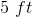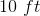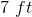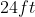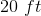Explanation:

Set up two equations from the given information: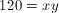and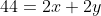Substitute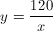into the second equation: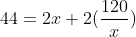Multiply through by.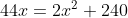Then divide by the coefficient of 2 to simplify your work: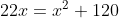Then since you have a quadratic setup, move the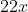term to the other side (via subtraction from both sides) to set everything equal to 0: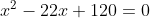As you look for numbers that multiply to positive 120 and add to -22 so you can factor the quadratic, you might recognize that -12 and -10 fit the bill. This makes your factorization: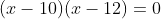This makes the possible solutions 10 and 12. Since 12 does not appear in the choices,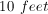is the only possible correct answer.

### Example Question #5 : Quadratic Equation

What is the sum of all the values ofthat satisfy: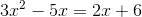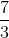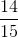Explanation:

With quadratic equations, always begin by getting it into standard form:Therefore, take our equation:And rewrite it as: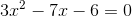You could use the quadratic formula to solve this problem.  However, it is possible to factor this if you are careful.  Factored, the equation can be rewritten as: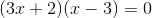Now, either one of the groups on the left could beand the whole equation would be.  Therefore, you set up each as a separate equation and solve for: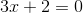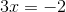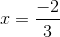OR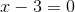The sum of these values is: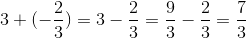### Example Question #1 : Quadratic Equation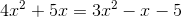Quantity A:Quantity B:A comparison cannot be detemined from the given information.

The two quantities are equal.

Quantity A is larger.

Quantity B is larger.

Quantity B is larger.

Explanation:

With quadratic equations, always begin by getting it into standard form: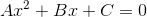Therefore, take our equation:And rewrite it as: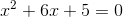Now, while you could use the quadratic formula to solve this problem, the easiest way to work this question is to factor the left side of the equation.  This gives you: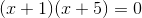Now, either one of the groups on the left could beand the whole equation would be.  Therefore, you set up each as a separate equation and solve for: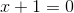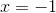OR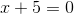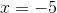Since both of your answers are less than, quantity B is larger than quantity A.

### Example Question #561 : Algebra

If f(x) = -x2 + 6x - 5, then which could be the value of a if f(a) = f(1.5)?

4.5
4
2.5
1
3.5
Explanation:

We need to input 1.5 into our function, then we need to input "a" into our function and set these results equal.

f(a) = f(1.5)

f(a) = -(1.5)2 +6(1.5) -5

f(a) = -2.25 + 9 - 5

f(a) = 1.75

-a2 + 6a -5 = 1.75

Multiply both sides by 4, so that we can work with only whole numbers coefficients.

-4a2 + 24a - 20 = 7

Subtract 7 from both sides.

-4a2 + 24a - 27 = 0

Multiply both sides by negative one, just to make more positive coefficients, which are usually easier to work with.

4a2 - 24a + 27 = 0

In order to factor this, we need to mutiply the outer coefficients, which gives us 4(27) = 108. We need to think of two numbers that multiply to give us 108, but add to give us -24. These two numbers are -6 and -18. Now we rewrite the equation as:

4a2 - 6a -18a + 27 = 0

We can now group the first two terms and the last two terms, and then we can factor.

(4a2 - 6a )+(-18a + 27) = 0

2a(2a-3) + -9(2a - 3) = 0

(2a-9)(2a-3) = 0

This means that 2a - 9 =0, or 2a - 3 = 0.

2a - 9 = 0

2a = 9

a = 9/2 = 4.5

2a - 3 = 0

a = 3/2 = 1.5

So a can be either 1.5 or 4.5.

The only answer choice available that could be a is 4.5.

### Example Question #2 : How To Find The Solution To A Quadratic Equation

Solve for x:  2(x + 1)2 – 5 = 27

–3 or 2

3 or 4

3 or –5

–2 or 4

–2 or 5

3 or –5

Explanation:

Quadratic equations generally have two answers.  We add 5 to both sides and then divide by 2 to get the quadratic expression on one side of the equation: (x + 1)2 = 16.   By taking the square root of both sides we get x + 1 = –4 or x + 1 = 4.  Then we subtract 1 from both sides to get x = –5 or x = 3.

### Example Question #1 : How To Find The Solution To A Quadratic Equation

Two consecutive positive multiples of three have a product of 54. What is the sum of the two numbers?

9

3

15

12

6

15

Explanation:

Define the variables to be x = first multiple of three and x + 3 = the next consecutive multiple of 3.

Knowing the product of these two numbers is 54 we get the equation x(x + 3) = 54. To solve this quadratic equation we need to multiply it out and set it to zero then factor it. So x2 + 3x – 54 = 0 becomes (x + 9)(x – 6) = 0.  Solving for x we get x = –9 or x = 6 and only the positive number is correct.  So the two numbers are 6 and 9 and their sum is 15.

### Example Question #1 : How To Find The Solution To A Quadratic Equation

Solve 3x2 + 10x = –3

x = –4/3 or –1

x = –1/6 or –6

x = –1/3 or –3

x = –2/3 or –2

x = –1/9 or –9

x = –1/3 or –3

Explanation:

First, the equations must be put in standard form: 3x2 + 10x + 3 = 0

Second, try to factor the quadratic; however, if that is not possible use the quadratic formula.

Third, check the answer by plugging the answers back into the original equation.

← Previous 1 3 4

Tired of practice problems?

Try live online GRE prep today.